# FARKAS MIKLÓS ALKALMAZOTT ANALÍZIS SZEMINÁRIUM ELŐADÁSAI 2017/18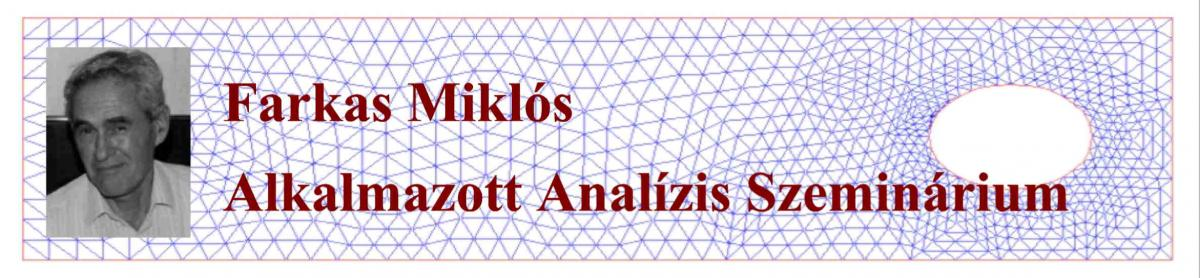3 May 2018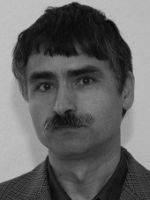Péter Ván (MTA Wigner Reseach Centre for Physics, BME Department of Energy Engineering)

Non-equilibrium thermodynamics and the origin of the evolution equations of continuum physics

In the theory of non-equilibrium thermodynamics, the entropy production is ​a ​derived ​quantity. According to the second law, its value cannot be negative. ​In classical non​-equilibrium ​thermodynamics this is the tool of the derivation of the Fourier-Navier-Stokes systems of equations, the constitutive equations for the material properties, and the evolution equations of the inner variables. In this talk, I will show how this method can be generalized and sharpened with stronger mathematical conditions and more accurate physical model constructions. I will show examples ​of higher order memory effects and weak nonlocality from the field of heat conduction and ​continuum mechanics. I will briefly ​​investigate the ​possibility of constructing symmetric ​hyperbolic ​partial differential equations​​ in this framework​.

26 April 2018Research reports of PhD students

10:15-10:30: Rahele Mosleh - Reliable numerical modelling of malaria propagation

10:30-10:45: Szilvia Császár -  Stability and exponential dichotomy

10:45-11:00: Bálint Takács - The SIR model with non-symmetric spatial dependence

11:00-11:15: Gábor Maros - Error estimates for a finite element approximation of fractional diffusion equations

11:15-11:30: Abdulla Obeidat - Operator splitting techniques for pricing American and European options

19 April 2018Gabriella Svantnerné Sebestyén (BME Department of Differential Equations)

Investigation of continuous and discrete population dynamics models

Population models represent an important field in Biomathematics. They give information and forecast about dynamics of the population. This talk will give an insight about population dynamics models in general and we will talk about two such models in detail. We will start with the stability analysis of the Easter Island population dynamics model than we will take a look at the numerical methods for the Lotka-Volterra system.

12 April 2018János Tóth (BME Department of Analysis)

On the Package ReactionKinetics.wl

When investigating the deterministic and/or stochastic models of chemical reactions a large amount of calculations have to be carried out. The preliminary steps are creating and studying different graphs describing reactions. This involves the use of combinatorics and linear algebra. One is also interested if mass is conserved in a model or not. This can be decided using the methods of linear programming. Stationary points and stationary distributions are to be determined which means the solution of large polynomial equations.  One has to solve (quite often: stiff) ordinary differential equations, simulate Markovian jump processes. Parameters of these processes are to be estimated based on measurements even in cases when not all the concentration time curves are known. This problem is implicit and highly nonlinear.  Our package gives help to all these tasks arising in chemistry (atmospheric chemistry), biochemistry (modeling metabolism), chemical engineering (combustion), but the models of chemical reaction kinetics are used outside chemistry, as well. We show the problems and solution methods from the point of programming and also a series of applications. A comparison with other programs will also be presented. A detailed description and instructions for use will be found in our book: J. Tóth, A. L. Nagy, D. Papp: Reaction Kinetics: Exercises, Programs and Theorems. (Mathematica for deterministic and stochastic kinetics), Springer, 2018 (to appear). (Joint talk with A. L. Nagy and D. Papp.)

22 March 2018Lajos Lóczi (ELTE IK Department of Numerical Analysis, BME Department of Differential Equations)

Step-size coefficients for boundedness of linear multistep methods

Monotonicity or boundedness properties (e.g. strong-stability-preserving, total-variation-diminishing or total-variation-boundedness properties) for linear multistep methods (LMMs) can be guaranteed by imposing step-size restrictions on the methods. To describe these restrictions, one introduces the concept of step-size coefficient for monotonicity (SCM, also referred to as the strong-stability-preserving (SSP) coefficient) and its generalization, the step-size coefficient for boundedness (SCB coefficient). A LMM with larger SCM or SCB is more efficient. The computation of the maximum SCM for a particular LMM is straightforward, however, it is more challenging to decide whether a SCB exists, or determine if a given number is a SCB. Based on some recent theorems in the literature we present methods to find the exact optimal SCB for a LMM. As an illustration, we consider SCBs in the BDF, extrapolated BDF, and Adams--Bashforth families.

8 March 2018Tamás Turányi (Institute of Chemistry, ELTE Eötvös Loránd University, Budapest)

Chemical Kinetic Optimization and  Uncertainty Quantification

Recently developed combustion models based on detailed reaction mechanisms may provide good results. The next question is how accurate the calculated results are, in other words, what is their uncertainty. The main source of uncertainty of the simulation results is the uncertainty of the chemical kinetic parameters. These parameters describe the temperature and pressure dependence of the rate coefficients. A first guess of the uncertainty of these parameters (“prior uncertainty”) may be based on directly measured rate coefficients and theoretical calculations. The temperature dependent uncertainty bands of the rate coefficients can be converted to the uncertainty domains of the Arrhenius parameters. Detailed reaction mechanisms can be developed and checked using the results of indirect measurements, like ignition delay time measurements in shock tubes and rapid compression machines, laminar burning velocity measurements, and concentration profile determinations in various reactors. At the optimization of detailed reaction mechanisms, all important rate parameters are fitted in one step within their domain of prior uncertainty using a global optimization method, taking into account all relevant indirect and direct measurements. The results of optimization are the set of the best estimated values of the important parameters that can be obtained from the experimental data considered, and also their covariance matrix, which is a good representation of their joint uncertainty. This posterior uncertainty domain is usually much smaller than the prior uncertainty one. The experimental data are suggested to be stored in ReSpecTh Kinetics Data Format files, allowing software independent and permanent storage. A series of software tools have been developed which allow the determination of prior and posterior uncertainty limits by processing ReSpecTh Kinetics Data Format files.

1 March 2018Gábor Kiss (Bolyai Institute, University of Szeged)

Rigorous numerics for periodic solutions to piecewise-linear delay equations

We present a novel computational tool for establishing the existence of periodic solutions of piecewise-linear delay differential equations. The method is based on the Chebyshev expansions of solutions. As an illustration, we use a piecewise-linear approximation of the celebrated Mackey-Glass equation.

22 February 2018Sándor Kovács (ELTE Department of Numerical Analysis, BME Department of Differential Equations)

Abstract bifurcations

Modelling of phenomena in applied sciences like physics, biology or engineering  often leads to equations depending on parameters. These parameters describe how the environment influences a system. Thus, the knowledge of the values of the parameters at which the qualitative behaviour of the system changes has great importance. In this talk we study the structure of  equations of the form $F(x,\lambda)=0,$ where $F$ is a nonlinear operator between Banach spaces and $\lambda$ represents the parameters.

15 February 2018

The Differential Equation that Solves Every Problem or How to Lie with Universal Equations

We present a new approach to the construction of first integrals for second order autonomous systems without invoking a Lagrangian or Hamiltonian reformulation. We show and exploit the analogy between integrating factors of first order equations and their Lie point symmetry and integrating factors of second order autonomous systems and their dynamical symmetry. We connect intuitive and dynamical symmetry approaches through one-to-one correspondence in the framework proposed for first order systems. Conditional equations for first integrals are written out, as well as equations determining symmetries. The equations are applied on the simple harmonic oscillator and a class of nonlinear oscillators to yield integrating factors and first integrals.

A Lie algebraic approach is also used to study universal equations. To be universal, a differential equation must have a solution with which an arbitrary function can be uniformly approximated. A universal differential equation must be form invariant under translation and scaling. We present a way to construct universal equations.

This is joint (not THAT type of joint) work with Sándor Balázs.

7 December 2017Research reports of PhD students

9:30-9:45: Rahele Mosleh - Mathematical models for malaria disease

9:45-10:00: Szilvia Császár -  Approximation of homoclinic orbits

10:00-10:15: Bálint Takács - Epidemic models with spatial dependence

10:15-10:30: Márton Neogrády-Kiss - Two simple models with inhibitory and excitatory neurons

10:30-10:45: Gábor Maros - Analysis of fractional diffusion problems

30 November 2017Yiannis Hadjimichael (BME Institute of Mathematics & MTA-ELTE NUMNET Research Group)

Optimal strong stability preserving time-stepping methods with upwind- and downwind-biased operators

A plethora of physical phenomena are modelled by hyperbolic partial differential equations, for which the exact solution is usually not known. Numerical methods are employed to approximate the solution to hyperbolic problems; however, in many cases, it is difficult to satisfy certain physical properties while maintaining high order of accuracy. Strong stability preserving (SSP) time discretizations were developed to ensure that nonlinear stability properties of the solution are maintained when coupled with suitable spatial discretizations.

In the first part of this talk, we review the development of optimal SSP Runge-Kutta and multistep methods for nonlinear problems. We emphasize the usage of an alternative representation of Runge-Kutta methods that reveals the SSP properties of such methods. Numerical examples illustrate the effectiveness and usefulness of SSP methods.
In the second part, we present some recent results related to perturbed methods that use both upwind- and downwind-biased spatial discretizations. We introduce a novel family of third-order implicit Runge–Kutta methods with arbitrarily large SSP coefficient and investigate the stability and accuracy of these methods. Moreover, we extend the analysis of SSP linear multistep methods to semi-discretized problems for which different terms on the right-hand side of the initial value problem satisfy different forward Euler (or circle) conditions. Optimal perturbed and additive monotonicity-preserving linear multistep methods are studied in the context of such problems.

23 November 2017Lubin G. Vulkov (Angel Kanchev University of Ruse, Bulgaria)

Adequate numerical methods for nonlinear parabolic problems in mathematical finance

The prices and hedging strategies in the real financial  market models are often described by fully nonlinear versions of the standard Black-Scholes equation. We concentrate on two classes of models: first, nonlinear Black-Scholes equations in which the volatility depends on  second space derivatives of the price(=solution) and then on regime-switching models described by systems of semilinear parabolic equations with exponential nonlinearities. The following characteristic  properties of these parabolic problems are typical: unbounded domain, boundary degeneration, maximum-minimum principle and nonnegativity preservation. We develop effective discretizations that reproduce these properties.

9 November 2017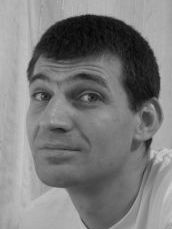Miklós Mincsovics (BME, Department of Differential Equations)

What is the difference between weakly and strongly stable linear multistep methods?

There are two main approaches to explain the differences between them. The first one relies on the role of the parasitic roots (this is what we usually teach). The second one is more indirect and based on the general definition of stability. Spijker was the first who presented a norm pair in which the midpoint method is not stable. This example can be extended to the general weakly stable case. Finally, we upgrade this latter approach keeping its advantages and eliminating its weak point.

26 October 2017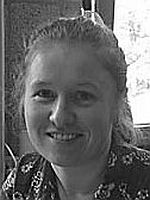Mónika Polner (Bolyai Institute, University of Szeged)

A space-time finite element method for neural field equations with transmission delays

Neural field equations are models that describe the spatio-temporal evolution of (spatially) coarse grained variables such as synaptic or firing rate activity in populations of neurons. We consider a single population of neurons, distributed over some bounded, connected, open region, whose state is described by their membrane potential. These potentials are assumed to evolve according to an integro-differential equation with space dependent delay.

Neural field models with transmission delay may be cast as abstract delay differential equations, which is the starting formulation for our numerical discretization. The numerical treatment of these systems is rare in the literature and has several restrictions on the space domain and the functions involved. The aim of this work is the development of an accurate numerical method without introducing limitations to its applicability. We present and analyze a novel time-discontinuous Galerkin finite element method. We give a theoretical analysis of the stability and order of accuracy of the numerical discretization and demonstrate the method on a number of neural field computations in one and two space dimensions.

19 October 2017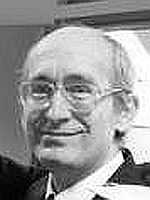Miklós Horváth (BME, Institute of Mathematics)

Inverse scattering: Mathematical properties of the phase shifts

The inverse scattering is one of the most useful devices to obtain information about quantum mechanical forces. The solutions of the radial Schrödinger equation, describing mathematically the situation, behave asymptotically like sine functions with shifted phase. The sequence of these phase shifts is a central notion in scattering theory. In this talk some classical and recent results about phase shifts will be presented and some open questions will be discussed.

12 October 2017Imre Fekete (Eötvös Loránd University & MTA-ELTE NUMNET)

On the zero-stability of multistep methods on smooth nonuniform grids

In order to be convergent, linear multistep methods must be zero stable. While constant step size theory was established in the 1950’s, zero stability on nonuniform grids is less well understood. Here we investigate zero stability on compact intervals and smooth nonuniform grids. The grid points are constructed as the image of an equidistant grid under a smooth deformation map. We show that for all strongly stable linear multistep methods, there is an $N^*$ such that a condition of zero stability is always fulfilled for $N > N^*$ under a smoothness condition. Examples are given for Adams and BDF type methods.

5 October 2017András Zachár (University of Dunaújváros, Institute of Informatics)

An explicit analytic solution of a coupled first order partial and ordinary differential equation system for a discontinuous initial-boundary value problem

Non-polynomial series solution of a coupled first order partial and ordinary differential equation (PDE-ODE) system for a discontinuous initial and boundary condition has been developed. Linear equation systems are constructed to calculate the constant coefficients of the series solution. Explicit expressions have been found to the solution of these linear equation systems. Different forms of the solution have been compared to the numerical solution of the PDE-ODE system and the rate of the convergence is also investigated. The studied first order PDE-ODE system describes an unsteady convection dominated heat transfer process induced by a buoyant plume entrainment.

28 September 2017Tamás Kalmár-Nagy

Devilish eigenvalues: hysteresis and mechanistic turbulence

We consider the adjacency matrix associated with a graph that describes transitions between 2^N states of the discrete Preisach memory model. This matrix can also be associated with the "last-in-first-out" inventory management rule. We present an explicit solution for the spectrum by showing that the characteristic polynomial is the product of Chebyshev polynomials. The eigenvalue distribution (density of states) is explicitly calculated and is shown to approach a scaled Devil's staircase. The eigenvectors of the adjacency matrix are also expressed analytically. This is joint work with Andreas Amann, Daniel Kim, and Dmitrii Rachinski. We also examine a mechanistic model of turbulence, a binary tree of masses connected by springs. We analyze the behavior of this linear model: a formula is presented for the analytical calculation of the eigenvalues and the optimal damping - at which the decay of the total mechanical energy is maximized. The discrete energy spectrum of the mechanistic model (defined as the total mechanical energy stored in each level) can be tuned to display the features of the Kolmogorov-spectrum. This is joint work with Bendegúz Dezső Bak.

21 September 2017István Faragó

Qualitatively reliable numerical models of time-dependent problems

In the modeling process we construct mathematical and numerical models. Both models should preserve the basic (physically, biologically, etc. motivated) qualitative properties of the original phenomena. In this talk this problem will be discussed. We examine the different qualitative properties (maximum principles, non-negativity preservation, maximum norm contractivity) for both models and we show the relation between them for the linear problems. For the numerical models we give the condition for the construction of the mesh under which the above qualitative properties are valid. The results will be demonstrated in different real-life problems. The main attention will be focused to the heat conduction problem. Briefly we discuss the compartmental epidemic models which take into the account the space dependence, and also some simple discrete Lotka-Volterra models.